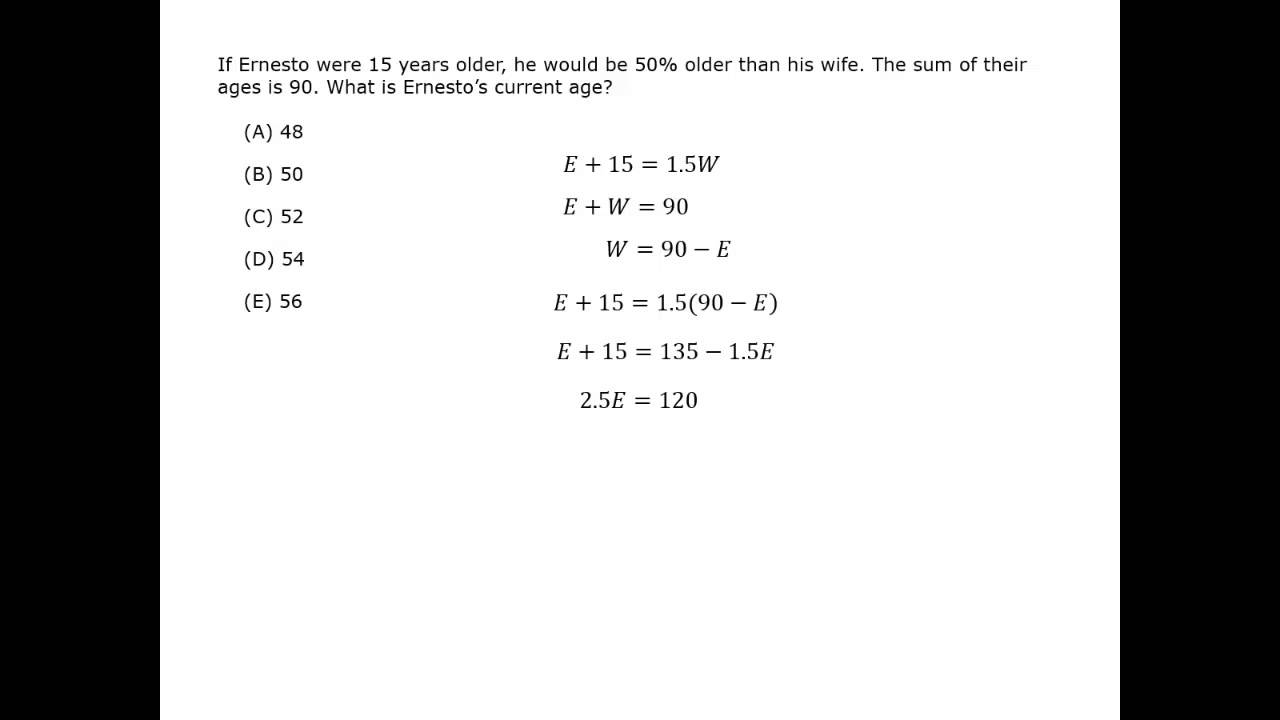Like all word problems, age problems require writing an equation using the words that define the relationships and actions. Age problems just use more of these words than other types of word problems.

The key is to calmly translate the words into manageable equations. Often each sentence requires a new equation.

The words that translate to the equals sign include “is,” “was” and “equals.”

Common words for other actions and relationships are defined in the following list.

 Example Word What to do? As equation The sum of John’s age and Steven’s is 19. sum addition j + s = 19 The difference between Todd’s age and his younger sister Sandra’s age is 5 years. difference subtraction (since Todd is older, it is Todd minus Sandra.) t – s = 5 The difference between Todd’s age and Sandra’s age is 5 years. difference subtraction with absolute value (you don’t know who is older) |t – s| = 5 John’s age is double Henry’s age. double multiplication j = 2h Four years ago, Kim was the same age as Bob is now. ago subtraction k – 4 = b Six less than my age is 22. less than subtraction a – 6 = 22 The total of Mario’s age and my age is 35. total addition m + a = 35 Ten more than my age equals 23. more than addition 10 + a = 23 Four times my age is 48. times multiplication 4a = 48 The product of my age and 12 is 144. times multiplication a × 12 = 144

Correctly translating the words into your own algebraic equation is critical, and it is easy to make mistakes. You can double check yourself by Plugging In your solution or Backsolving from the answer choices.

Steven is 12 years older than Mary. Three years ago, Steven was 5 times as old as Mary.
How old is Mary?

### Solution

Define the variables.

Mary’s age = m
Steven’s age = s

There are 2 variables, so you need 2 equations.

Sentence                                                           Equation

Steven is 12 years older than Mary.               s = m + 12

Three years ago, Steven was 5 times as old as Mary. s – 3 = 5(m – 3)
Simplify the equation.                                                       s – 3 = 5m – 15
s = 5m – 12

Use the 2 equations and solve.

s = m + 12 and s = 5m – 12
m + 12 = 5m – 12
4m = 24
m = 6 Mary is 6 years old.

Ethan is as much older than Harry as Harry is older than Candice. Five years ago, Ethan’s age was double what the age difference between what his age and Harry’s will be 15 years from now.
How old is Candice?

### Solution

Define the variables.

Ethan’s age = e
Harry’s age = h
Candice’s age = c

There are 3 variables, but you only need to solve for one variable, c. So you need 3 or possibly just 2 equations.

Sentence
Ethan is as much older than Harry as Harry is older than Candice.
Simplify the equation.

Break down each phrase of the next sentence.
Five years ago, Ethan’s age
was
double
difference between
what Ethan’s age and Harry’s will be 15 years from now

Write the complete equation.
Simplify the equation.

Equation
e h = h c
e = 2hc

e – 5
=
× 2
– (subtraction)
e + 15 and h + 15
e – 5 = 2[(e + 15) – (h + 15)]

e – 5 = 2[e + 15 – h – 15]
e – 5 = 2[eh]
e – 5 = 2e – 2h
e = 2h – 5

Use the 2 equations and solve.

e = 2hc
e = 2h – 5

2hc = 2h – 5

c = 5 Candice is 5 years old.

In this case you only need two equation

Before attempting these problems, be sure to review this section on data sufficiency questions.

#### Age

Best viewed in landscape mode

2 questions with video explanations

100 seconds per question